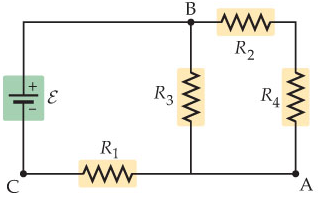# Problem: Consider the circuit shown below. Suppose four resistors in this circuit have the values of R1 = 13 Ω, R2 = 7.5 Ω, R3 = 7.7 Ω and R4 = 10 Ω, and that the emf of the battery is 18 V Find the current through each resistor using Kirchoff's rules

###### FREE Expert Solution

Equivalent resistance for resistors in parallel for 2 resistors:

$\overline{){{\mathbf{R}}}_{{\mathbf{eq}}}{\mathbf{=}}\frac{{\mathbf{R}}_{\mathbf{1}}{\mathbf{R}}_{\mathbf{2}}}{{\mathbf{R}}_{\mathbf{1}}\mathbf{+}{\mathbf{R}}_{\mathbf{2}}}}$

Equivalent resistance for resistors in series:

$\overline{){{\mathbf{R}}}_{{\mathbf{eq}}}{\mathbf{=}}{{\mathbf{R}}}_{{\mathbf{1}}}{\mathbf{+}}{{\mathbf{R}}}_{{\mathbf{2}}}{\mathbf{+}}{\mathbf{.}}{\mathbf{.}}{\mathbf{.}}{\mathbf{+}}{{\mathbf{R}}}_{{\mathbf{n}}}}$

Current:

$\overline{){\mathbf{i}}{\mathbf{=}}\frac{\mathbf{V}}{\mathbf{R}}}$

Kirchoff's junction rule:

$\overline{){\mathbf{\Sigma }}{\mathbf{i}}{\mathbf{=}}{\mathbf{0}}}$

Kirchoff's loop rule:

$\overline{){\mathbf{\Sigma V}}{\mathbf{=}}{\mathbf{0}}}$

where V = iR

R2 and R4 are in series.

R24 = 7.5 + 10 = 17.5 Ω

R24 and R3 are in parallel.

R234 = (17.5)(7.7)/(17.5 + 7.7) = 5.35 Ω

R1 and R234 are in series.

Req = R1234 = 13 + 5.35 = 18.35Ω

Current, i = 18/18.35 = 0.981 A

84% (107 ratings)###### Problem Details

Consider the circuit shown below. Suppose four resistors in this circuit have the values of R1 = 13 Ω, R2 = 7.5 Ω, R3 = 7.7 Ω and R4 = 10 Ω, and that the emf of the battery is 18 VFind the current through each resistor using Kirchoff's rules